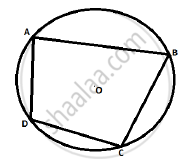# Prove that ‘The Opposite Angles of a Cyclic Quadrilateral Are Supplementary’. - Geometry

Sum

Prove that ‘the opposite angles of a cyclic quadrilateral are supplementary’.

#### Solution

To prove:- ∠BAD + ∠BCD = 180º

and ∠ABC + ∠ADC = 180ºProof :-

Arc BCD is intercepted by the inscribed ∠BAD.

therefore angle"BAD"=1/2"m"("arc BCD")..........(1)

(Inscribed angle theorem)

Arc BAD is intercepted by the inscribed ∠BCD.

therefore angle"BCD"=1/2"m" ("arc DAB")..........(2)

(Inscribed angle theorem)

From (1) and (2) we get

∠BAD + ∠BCD = 1/2[M(arc BCD) + M(arc DAB)]

= (1/2)*360°

= 180°

Again, as the sum of the measures of angles of a quadrilateral is 360°.

= 360° - 180°

= 180°

Hence the opposite angles of a cyclic quadrilateral are supplementary.